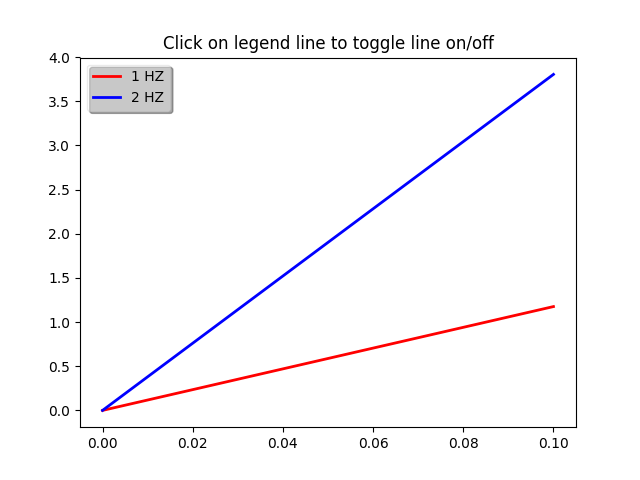# Legend Picking¶

Enable picking on the legend to toggle the original line on and offimport numpy as np
import matplotlib.pyplot as plt

t = np.arange(0.0, 0.2, 0.1)
y1 = 2*np.sin(2*np.pi*t)
y2 = 4*np.sin(2*np.pi*2*t)

fig, ax = plt.subplots()
ax.set_title('Click on legend line to toggle line on/off')
line1, = ax.plot(t, y1, lw=2, color='red', label='1 HZ')
line2, = ax.plot(t, y2, lw=2, color='blue', label='2 HZ')
leg = ax.legend(loc='upper left', fancybox=True, shadow=True)
leg.get_frame().set_alpha(0.4)

# we will set up a dict mapping legend line to orig line, and enable
# picking on the legend line
lines = [line1, line2]
lined = dict()
for legline, origline in zip(leg.get_lines(), lines):
legline.set_picker(5)  # 5 pts tolerance
lined[legline] = origline

def onpick(event):
# on the pick event, find the orig line corresponding to the
# legend proxy line, and toggle the visibility
legline = event.artist
origline = lined[legline]
vis = not origline.get_visible()
origline.set_visible(vis)
# Change the alpha on the line in the legend so we can see what lines
# have been toggled
if vis:
legline.set_alpha(1.0)
else:
legline.set_alpha(0.2)
fig.canvas.draw()

fig.canvas.mpl_connect('pick_event', onpick)

plt.show()


Keywords: matplotlib code example, codex, python plot, pyplot Gallery generated by Sphinx-Gallery